# Vacuum Tube Amplifier Circuits -- BASIC VACUUM-TUBE ACTIONS

 An elementary understanding of the actions within a vacuum tube is essential before those which occur outside the tube can be understood. Most of this guide is devoted to a study of common circuit actions which are regulated in some manner within the tube. These basic phenomena, which occur over and over in the operation of any tube include: 1. Free electron emission from a heated cathode. 2. Easy passage of free electrons through a vacuum. 3. Attraction of electrons to positive voltage points. 4. Repulsion of electrons from negative voltage points. DIODE TUBES The diode is the earliest, and also the simplest, vacuum tube. The discovery of the Edison effect by Thomas Alva Edison led to the invention of the diode. Edison had observed that when one of two conductors terminated inside an evacuated glass envelope was heated, a small electric current would flow between the conductors under certain other conditions of applied voltage. Al though he recorded his observations, he made no immediate at tempt to explain or apply them. The phenomenon he observed can be fully explained by examining the operation of the diode vacuum tube. Fig. 1 reveals its essential construction details in symbolic form. The two elements, called electrodes, are the cathode and the plate (sometimes referred to as the anode). Three conditions must be met in order for electric current (meaning electrons) to flow across the evacuated space between the cathode and plate of the tube: 1. The cathode must be made hot enough that free electrons will actually "jump" off the cathode. 2. A positive voltage must be applied to the plate in order to attract the negative electrons which have left the cathode. 3. The space between the electrodes must be evacuated of all air molecules, so that there will be nothing within the tube to hinder the movement of electrons. The relative ease or difficulty with which electrons will leave the heated cathode is called the work function of the cathode material. When any material is heated, a tremendous increase in molecular motion occurs. This molecular agitation results in the releasing, or "shaking off," of many of the electrons from their orbit around the nuclei of the various molecules. Once it is re leased from its planetary orbit within a molecule, such an electron becomes what is known as a "free" electron. Some electrons from the molecules immediately adjacent to the surface will not only jump free of their parent molecules, but may jump free of the surface of the metal also. Any electron which has done the latter becomes available for making up space current (the name applied to the electron current drawn across vacuum tubes, from cathode to plate). This process of releasing electrons into the tube by heating the cathode can be likened to the familiar pot of boiling water. If you observe the boiling water closely, you will be able to see tiny droplets jumping off its surface. This analogy gives rise to the fairly common expression that electrons "boil off the cathode." Gravity normally causes the droplets of water to fall back onto the surface. Electrons, however, do not respond to gravitational force but to electric forces. Since all electrons carry one negative electric charge, they are repelled by each other, and hence by any negative voltage, however small. By the same token, they are all attracted by positive voltages. It thus becomes a simple matter to attract electrons across the vacuum tube by applying a suit able positive voltage to the plate. The amount of positive voltage determines to some extent the number of electrons that will cross the tube, and the size and shape of the cathode itself is another factor.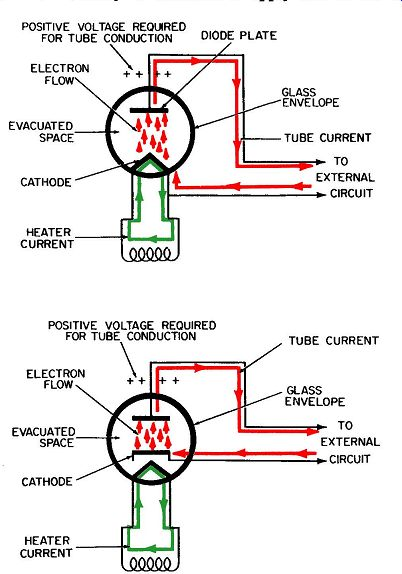Fig. 1. The diode vacuum tube. Fig. 2 shows a common graphical representation of the volt age-current characteristic of a 2X2A diode tube. A curve of this nature reveals the amount of current that will cross the tube for any particular plate voltage. As an example, a plate voltage of 100 volts (which is read on the horizontal scale) indicates that approximately 15 milliamperes of current will flow across the tube ( this being read on the vertical scale as shown by the dashed line) . Another dashed line shows that if 200 volts is applied to the plate, about 35 milliamperes of current will flow. Literally hundreds of different diode tubes are available, all operating in the same fundamental fashion described. These tubes will differ in construction, depending on the amount of plate voltage it is necessary or desirable to apply and on the amount of plate current (tube current) desired. The amount of current required is dictated by external circuit considerations, some of which are discussed in later Sections. Diode vacuum tubes are used extensively as rectifiers and detectors. The term rectifier is usually applied to a circuit for converting low-frequency alternating current into direct current. The term detector, on the other hand, is usually applied to a circuit employed to demodulate a high-frequency (usually a radio frequency) alternating current. That is, a detector separates the low-frequency component of a signal from the high-frequency component it is superimposed on. A detector cannot demodulate without first rectifying the alternating current. Therefore, only unidirectional currents will flow as a result of this rectifying action. (A unidirectional current is one that, although varying in amplitude, always flows in one direction.)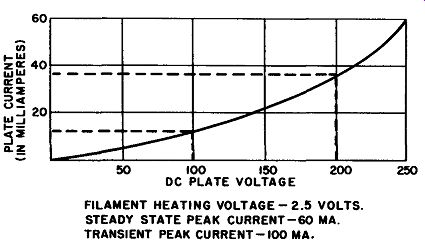Fig. 2. Plate voltage-current characteristics of a typical diode TRIODE TUBES Fig. 3 shows the commonly accepted symbol for a triode vacuum tube. The triode differs from the diode in that a third element, called the control grid, has been added between the cathode and plate. The control grid is constructed of very fine mesh wire, and because it is between the cathode and plate, any electrons which flow from one to the other must pass through the mesh itself. The control grid is usually much closer to the cathode than to the plate. The wire-mesh construction and this closeness to the cathode make it possible to regulate, or control, the electron stream through the tube by varying the voltage of the control grid. The control grid, invented early in the twentieth century by Dr. Lee de Forest, made it possible to amplify small signals perhaps the most important single function performed by electronic circuitry. Tubes used as amplifiers are so constructed that a small charge in voltage at the grid will cause a relatively large change in the current flowing through the tube. The changes in tube current flowing through the external load will then develop a correspondingly large variation in the voltage present at the plate of the tube. To understand how a tube amplifies, it is necessary for you to understand its characteristic curves. There are three quantities in question, and a change in any one will affect the amount of amplification attainable. These three quantities are the grid volt age, plate voltage, and plate current. (The term grid voltage is sometimes taken to mean the difference in voltage between the grid and cathode. However, a more correct term for this voltage is grid bias. If the cathode is maintained at zero voltage (ground), then the actual voltage at the grid is also the grid bias. However, when the cathode is maintained at some other voltage, as is more frequent, the voltage at the cathode must be subtracted from the voltage at the grid to arrive at the proper "grid voltage" to be used with the characteristic curves of the tube.) Fig. 4 shows the plate characteristic curves for a 6BN4. This is a triode tube which is used primarily as an RF amplifier in television receivers. The abscissa, or horizontal scale, is measured in volts and represents the possible range over which the plate voltage of the tube may be varied. The ordinate, or vertical scale, is measured in milliamperes and represents the range over which the plate current will vary for the various grid voltages during operation. The curves across the face of the graph represent particular values of grid voltage (grid bias) which is designated as Ee. The curve farthest to the left represents a grid voltage of zero. Each point on this line tells us how much plate current will flow through this tube, for the particular value of plate voltage, when the grid voltage is zero. As an example, the vertical line which represents 100 volts of plate voltage intersects the zero grid-voltage curve at point A. The dashed horizontal line, which also intersects point A, indicates that approximately 19 milliamperes of plate current will flow through the tube for this combination of grid and plate volt ages. As long as the combination of O volts on the grid and 100 volts on the plate is maintained, 19 milliamperes of current will flow through the tube. Voltage amplification cannot be demonstrated adequately from a single example. By taking a second example, however, we can come closer to describing its meaning. Consider now what would happen if we should change the grid voltage from zero to -1 volt, without changing the plate voltage. The grid-voltage line for -1 volt intersects the plate voltage line for 100 volts at point B. Reading horizontally from point B to the left edge of the graph, we see that approximately 9 milliamperes of plate current will flow through the tube for this new combination of grid and plate voltages.Fig. 3. The triode vacuum tube. The most important point to grasp from these two examples is this-by reducing the grid voltage (meaning to make it more negative) , we can reduce the amount of plate current which flows through the tube. By the same token, when we increase the grid voltage (meaning to make it less negative--or more positive), we can increase the amount of current through the tube. These two examples enable us to arrive at a definition of the mutual transconductance (g111 ) of a tube. Mutual transconductance is defined as the ratio between a small change in the plate current and the corresponding change in grid voltage which caused it, when the plate voltage is maintained constant. Transconductance (the name it usually goes by) is measured in mhos or micromhos. (From Ohm's law we know that the ratio of volt age to current is a measure of resistance, which is measured in ohms. Therefore, since transconductance is just the opposite--the ratio of current to voltage--it has been given the unit of measurement of a mho which is ohm spelled backwards.) Since plate current is normally measured in milliamperes, it becomes more convenient to measure transconductance in millionths of a mho, or micromhos. Values of several thousand micro mhos are normal; the 6BN4 tube used in this example has a trans conductance of 7700 micromhos. Many tube testers have their meters calibrated directly in micromhos. One of the commonest symptoms of vacuum-tube failure is a decrease in the transconductance; this automatically implies a decrease in the total emission of electron current within the tube.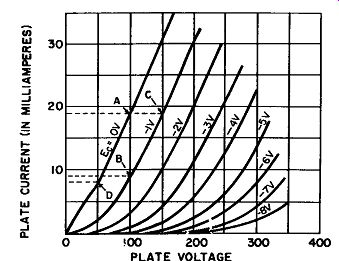Fig. 4. Plate characteristic curves of a typical triode. The second important rating factor of vacuum tubes is the amplification factor. It is the ratio between changes in grid and plate voltages, when the plate current is maintained constant. Fig. 4 can also be used to describe this rating factor. Suppose a line is drawn horizontally through point A until it intersects the next grid line, which is for a grid voltage of -1 volt. The point of inter section with this grid-voltage line has been labeled point C. It is evident, from the bottom scale for plate voltage, that point C represents a plate voltage of 150 volts. This is 50 volts greater than is represented by point A. Thus, a 1 volt change in grid voltage corresponds to, or has the same effect on plate current as, a 50-volt change in plate voltage. The amplification factor is the ratio of these two amounts, or: 50 volts/1 volt = 50 In other words, a tube with these values is said to have an amplification factor (µ. or mu) of 50. This value of mu tells us the grid voltage is 50 times more effective than the plate voltage in con trolling the flow of plate current. The third important rating factor for vacuum tubes is a mythical quantity known as the plate resistance, the symbol for which is rp. It is the resistance (in ohms) of the path between the cathode and the plate of the tube, which, of course, is not measurable with a meter. It is equal to the ratio between a small change in plate voltage and the corresponding change in plate current, when the grid voltage is maintained constant. This can also be understood from Fig. 4. The two points used to illustrate this quantity are A and D, both of which are on the same zero grid-voltage line. The change in plate current between these two points is evidently 19 - 8, or 11, milliamperes. This is read from the vertical scale at the left. The change in plate voltage, read from the horizontal scale at the bottom, is evidently 100 - 50, or 50 volts. These two quantities enable us to compute an approximate value of plate resistance for the particular operating conditions, as follows: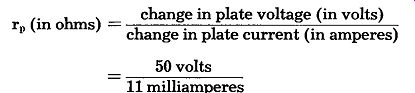... converting milliamperes to amperes, we have 50 rp= .011 rp = 4545 ohms Because of the curvature of the grid-voltage lines in Fig. 4, the locations of points A and subsequently B, C, and D will have some bearing on the exact amounts calculated for the three quantities. If point A had initially been positioned on a different grid voltage line ( or for a different combination of plate current and plate voltage) , the changes in these quantities-represented by moving to points B, C, and D-will of course be different. Like wise, the calculated values of transconductance, amplification factor, and plate resistance will be changed somewhat. Each of these quantities--mu, gm, and rp--is of great significance in the mathematics of electronic tube actions. (These mathematical considerations are beyond the scope of this guide.) Transconductance (gm) probably has the greatest everyday significance to the technician, since some tube testers are calibrated directly in transconductance. Also, any decrease below a specified level will instantly identify tubes which have "failed" ( cannot deliver the proper amount of electron current from cathode to plate). TETRODE TUBES A refinement in vacuum-tube functions is made possible by the addition of a second grid between the control grid and plate as shown in Fig. 5. This screen grid, as it is called, is also a fine wire mesh. All electron current which eventually reaches the plate must pass through the grid wires. The screen grid has two important functions: 1. Its screening action between the plate and control grid serves to isolate the voltage changes at the plate from those at the control grid, thereby preventing undesirable feedback be tween plate and grid. 2. It speeds up, or "accelerates," the plate-current stream flowing through the tube. In this way, electrons are prevented from accumulating around the cathode and impeding the plate-current flow. This accumulation is called space charge. In addition, the amount of plate current flowing is almost independent of the plate voltage, thus making a higher amplification factor possible. To understand the screening action, it is first necessary for you to understand the nature and effects of interelectrode capacitance between the plate and control grid. In a tube, these two elements act like plates of a capacitor ( capacitor action is covered in sub sequent Sections). Suffice it to say here that whenever a radio frequency voltage exists in the plate circuit, a radio-frequency current at the same frequency will also be present. During each cycle this current will drive a small quantity of electrons on and off the plate. As electrons are driven onto the plate, from its external circuit, an equal number will be driven off the control grid and into the grid circuit. Likewise, as electrons are drawn off the plate, an equal number of electrons will be drawn onto the grid from its external circuit. Thus, this feedback action induces an RF current/voltage combination in the grid circuit, with results that are generally undesirable. Primarily because it is closer to the plate than to the control grid, the screen grid will exhibit much more capacitance to the plate. Consequently, it acts as an electrostatic shield between the plate and control grid. That is, radio-frequency voltages at the plate will in effect be isolated from the control grid. As a result, a similar RF current/voltage combination will be set up in the screen-grid circuit, where it can easily be diverted, or bypassed, to ground.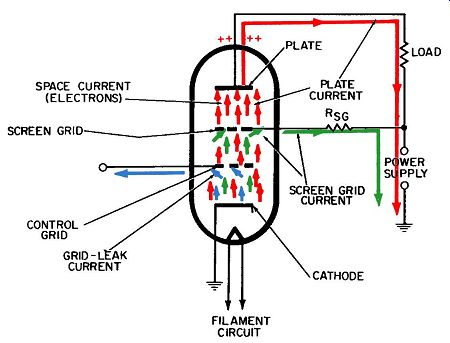Fig. 5. The tetrode vacuum tube. The screen grid normally is connected to a B+ supply voltage, whit is about the same value as the plate voltage; or through a resistor, which results in a somewhat lower screen voltage. Be cause it is between the plate and the cathode, and has a high positive voltage on it, the screen grid provides an extra attraction to the electrons leaving the cathode. Thus, they are drawn away from the cathode area more easily, and in greater quantity, than if the screen grid were not there. PENTODE TUBES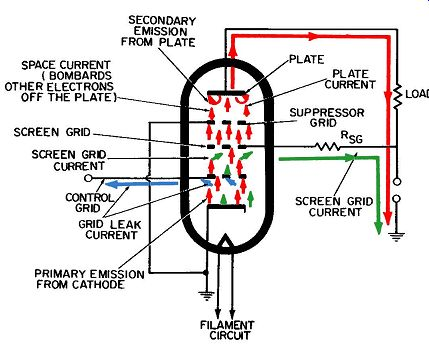Fig. 6. The pentode vacuum tube. Fig. 6 shows the conventional symbol for the five-element tube called the pentode. (The filament and the connecting pins to it, required for heating the cathode, are not considered elements.) The pentode tube has three different grids between its cathode and plate. In addition to control and screen grids, ( the functions of which were described for triode and tetrode tubes), the pentode has a third grid, called the suppressor grid, between the screen and plate. Recall that when a high positive voltage is applied to it, the screen grid draws the electron stream toward it from the cathode, at a much higher velocity than if there were no screen grid. Most of this electron stream passes through the mesh of the screen grid and is then in position to be attracted by the high positive voltage of the plate. Traveling at such a high velocity, these electrons will bombard the plate and discharge, or "knock off," other "secondary" electrons. Once they have entered the open space between plate and screen grid, these secondary electrons are subjected to the positive voltages of the screen grid and plate. Under these conditions, a large percentage of the secondary electrons would probably be attracted to the screen grid and exit from the tube via it. This phenomenon of electrons being knocked off the plate and re-entering the electron stream is called secondary emission. It is disadvantageous in two ways: 1. It will increase the total amount of screen-grid current, but in such an unpredictable manner that precise operation of the tubes and their circuits is very difficult. 2. It will decrease the total plate current by an equal amount and thereby largely nullify one of the principal reasons for adding a screen grid in the first place-namely, to increase the amount of plate current through the tube. These disadvantages are circumvented by the suppressor grid between the screen and plate. The prime function of this grid is to suppress secondary emission of electrons from the plate of the tube. It is usually connected to the cathode and exhibits its same low voltage. Secondary electrons from the plate, upon entering the space between the plate and suppressor grid, will now "see" this low voltage instead of the high positive voltage of the screen grid. Therefore, the high positive voltage on the plate will exhibit a much stronger attraction for these electrons than the low suppressor grid voltage. As a result, most of them will return to the plate. The plate-current stream must of course pass through the wire mesh of the suppressor grid. However, the electrons in this stream will be relatively unaffected by the low voltage on the suppressor grid, because their own inertia carries them beyond it. Once these electrons have moved beyond the suppressor grid, the high positive plate voltage quickly draws them to the plate.
Updated: Monday, 2020-09-07 16:15 PST